#There are 366 different Starters of The Day, many to choose from. You will find below some starters on the topic of Investigations. A lesson starter does not have to be on the same topic as the main part of the lesson or the topic of the previous lesson. It is often very useful to revise or explore other concepts by using a starter based on a totally different area of Mathematics.

Main Page

### Investigations Starters: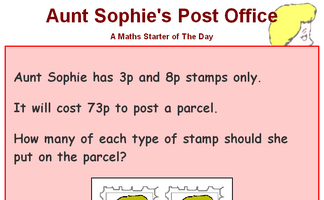Work out the number of stamps needed to post a parcel.How many different ways can four people stand in line?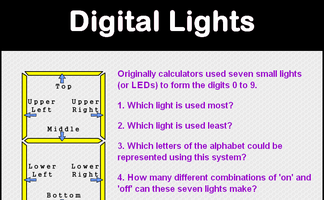Questions about the Small LEDs used to make up the digits on a calculator display.Use the flowchart to generate a sequence of numbers. Which number will reach 1 the fastest?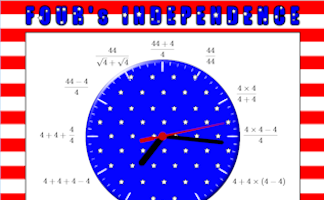A clock face containing only the number 4. Can you make a clock face containing any other single number?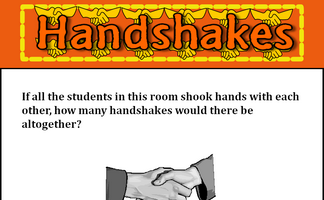If all the students in this room shook hands with each other, how many handshakes would there be altogether?To find out whether a number is happy or not, square each of its digits, add the answers and repeat. If you end up with 1 the number is happy! How many other happy numbers can you find?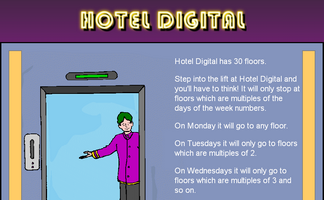A puzzle about the lifts in a hotel which serve floors based on the day of the week.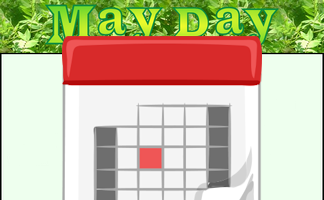Add together the dates of all the Thursdays in May this year. Which day sum is largest?Which numbers when multiplied by the number of letters in the word(s) of the number give square numbers?Arrange the numbers 1-9 to make three 3 digit numbers that add up to 999.Investigate three fractions which add together to give one fifth.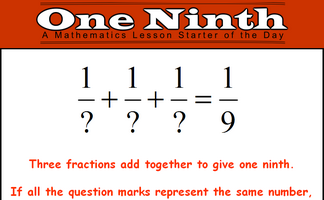Investigate three fractions which add together to give one ninth.Find other numbers that can be changed to 1 on a calculator using only the 4 key and any operation.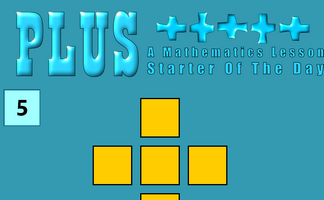A number puzzle suitable for children with a wide range of abilities.Calculate the areas of all the possible quadrilaterals that can be constructed by joining together dots on this grid.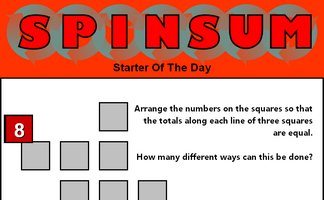Arrange the numbers on the grid of squares so that the totals along each line of three squares are equal.Place the numbers on the triangle so that the totals along each of the sides are equal.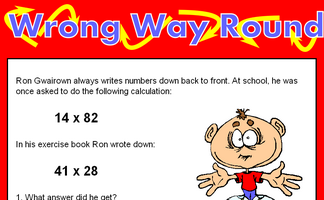Find calculations which written back to front give the same answer.

### Search

The activity you are looking for may have been classified in a different way from the way you were expecting. You can search the whole of Transum Maths by using the box below.

Have today's Starter of the Day as your default homepage. Copy the URL below then select
Tools > Internet Options (Internet Explorer) then paste the URL into the homepage field.

Set as your homepage (if you are using Internet Explorer)

Do you have any comments? It is always useful to receive feedback and helps make this free resource even more useful for those learning Mathematics anywhere in the world. Click here to enter your comments.For All: